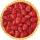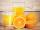# Determine the number

Determine the number x thatisfrom one half from.

Result

x =  2.23

#### Solution:Leave us a comment of example and its solution (i.e. if it is still somewhat unclear...):Be the first to comment!#### To solve this example are needed these knowledge from mathematics:

Need help calculate sum, simplify or multiply fractions? Try our fraction calculator. Do you have a linear equation or system of equations and looking for its solution? Or do you have quadratic equation?

## Next similar examples:

1. Unknown numberIdentify unknown number which 1/5 is 40 greater than one tenth of that number.
2. FractionsThree-quarters of an unknown number are 4/5. What is 5/6 of this unknown number?
3. Unknown numberI think the number - its sixth is 3 smaller than its third.
4. UN 1If we add to an unknown number his quarter, we get 210. Identify unknown number.
5. EquationSolve the equation: 1/2-2/8 = 1/10; Write the result as a decimal number.
6. Strange xFor what x is true ??
7. Cleaning windowsCleaning company has to wash all the windows of the school. The first day washes one-sixth of the windows of the school, the next day three more windows than the first day and the remaining 18 windows washes on the third day. Calculate how many windows ha
8. PearsThere were pears in the basket, I took two-fifths of them, and left six in the basket. How many pears did I take?
9. EqnSolve equation with fractions: 2x/3-50=40+x/4
10. Equation with xSolve the following equation: 2x- (8x + 1) - (x + 2) / 5 = 9
11. Simple equation 6Solve equation with one variable: X/2+X/3+X/4=X+4
12. Equation with fractionsSolve equation: ? It is equation with fractions.
13. TripOn the trip drank 3/10 of pupils tea, 2/5 cola, 1/4 mineral water and remaining 3 juice. How many students were on the trip?
14. TogetherGrandfather, father, and son are carpenters. They decided to make a wardrobe. Father produces it for 12 hours, his son for 15 hours, and grandfather for 10 hours. How long will they make it together?
15. Class 8.AThree quarters of class 8.A went skiing. Of those who remained at home one third was ill and the remaining six were on math olympic. How many students have class 8.A?
16. SeamstressThe seamstress cut the fabric into 3 parts. The first part was the eighth fabric, the second part was three-fifths of the fabric and the third part had a length of 66 cm. Calculate the original length of the fabric.
17. Simple equationSolve for x: 3(x + 2) = x - 18